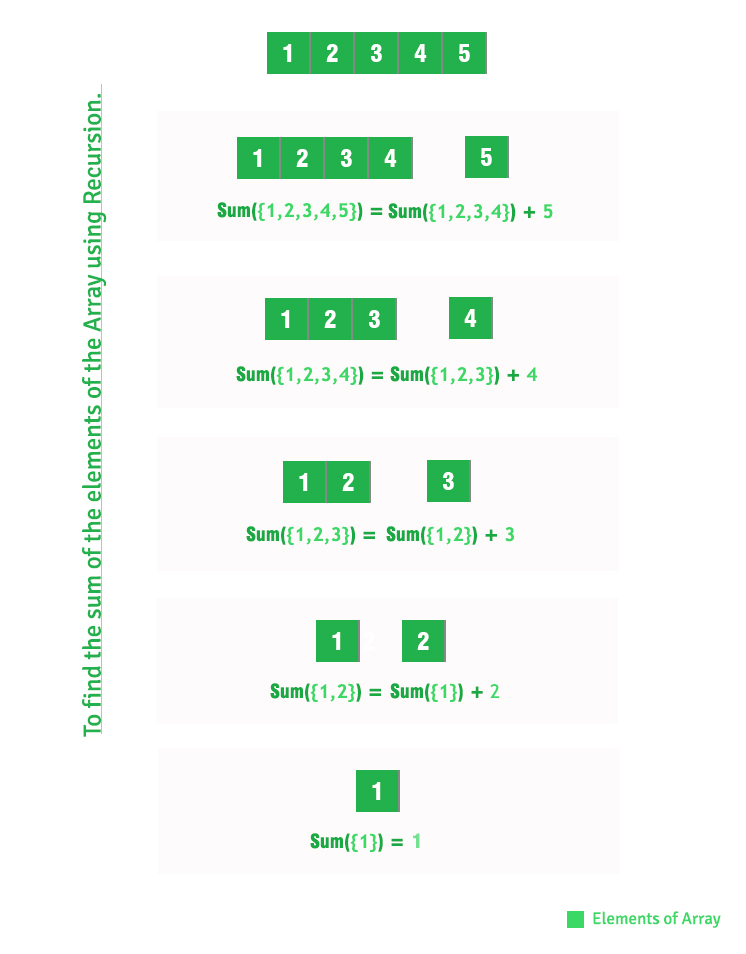Related Articles

# Sum of array elements using recursion

• Difficulty Level : Basic
• Last Updated : 23 Jun, 2021

Given an array of integers, find sum of array elements using recursion.
Examples:

```Input : A[] = {1, 2, 3}
Output : 6
1 + 2 + 3 = 6

Input : A[] = {15, 12, 13, 10}
Output : 50```

We have discussed iterative solution in below post.
Sum of elements in a given array
In this post, recursive solution is discussed.

## C++

 `// C++ program to find sum of array``// elements using recursion.``#include ` `// Return sum of elements in A[0..N-1]``// using recursion.``int` `findSum(``int` `A[], ``int` `N)``{``    ``if` `(N <= 0)``        ``return` `0;``    ``return` `(findSum(A, N - 1) + A[N - 1]);``}` `// Driver code``int` `main()``{``    ``int` `A[] = { 1, 2, 3, 4, 5 };``    ``int` `N = ``sizeof``(A) / ``sizeof``(A);``    ``printf``(``"%dn"``, findSum(A, N));``    ``return` `0;``}`

## Java

 `// Java program to find sum of array``// elements using recursion.` `class` `Test {``    ``static` `int` `arr[] = { ``1``, ``2``, ``3``, ``4``, ``5` `};` `    ``// Return sum of elements in A[0..N-1]``    ``// using recursion.``    ``static` `int` `findSum(``int` `A[], ``int` `N)``    ``{``        ``if` `(N <= ``0``)``            ``return` `0``;``        ``return` `(findSum(A, N - ``1``) + A[N - ``1``]);``    ``}` `    ``// Driver method``    ``public` `static` `void` `main(String[] args)``    ``{``        ``System.out.println(findSum(arr, arr.length));``    ``}``}`

## Python

 `# Python program to find sum of array``# elements using recursion.` ` ``# Return sum of elements in A[0..N-1]`` ``# using recursion.``  ``def` `_findSum(arr, N):``     ``if` `len``(arr)``=``=` `1``:``        ``return` `arr[``0``]``     ``else``:``        ``return` `arr[``0``]``+``_findSum(arr[``1``:], N)` `# driver code``arr ``=``[]``# input values to list``arr ``=` `[``1``, ``2``, ``3``, ``4``, ``5``]`` ` `# calculating length of array``N ``=` `len``(arr)`` ` `ans ``=``_findSum(arr,N)``print` `(ans)`` ` `# This code is contributed by Khare Ishita`

## C#

 `// C# program to find sum of array``// elements using recursion.``using` `System;` `class` `Test {``    ` `    ``static` `int` `[]arr = {1, 2, 3, 4, 5};` `    ``// Return sum of elements in``    ``// A[0..N-1] using recursion.``    ``static` `int` `findSum(``int` `[]A, ``int` `N)``    ``{``        ``if` `(N <= 0)``            ``return` `0;``        ``return` `(findSum(A, N - 1) + A[N - 1]);``    ``}` `    ``// Driver Code``    ``public` `static` `void` `Main()``    ``{``        ``Console.Write(findSum(arr, arr.Length));``    ``}``}` `// This code is contributed by Nitin Mittal.`

## PHP

 ``

## Javascript

 ``

Output:

`15`

How does above recursive solution work?This article is contributed by Prakhar Agrawal. If you like GeeksforGeeks and would like to contribute, you can also write an article using write.geeksforgeeks.org or mail your article to review-team@geeksforgeeks.org. See your article appearing on the GeeksforGeeks main page and help other Geeks.